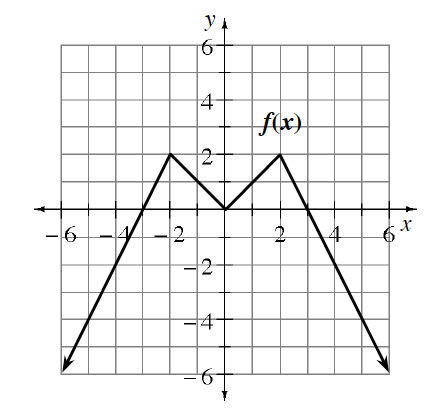### Home > PC3 > Chapter 7 > Lesson 7.2.2 > Problem7-92

7-92.

Given the graph of $y=f(x)$ at right, sketch graphs of the following transformations.

1. $y=-f(x)+2$

Reflect the curve vertically, then shift it up $2$ units.

2. $y=\frac{1}{2}f(x+2)$

Shift the curve left $2$ units, then halve the $y\text{-values}$.